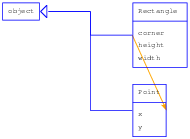# 14.5: Class DiagramsFigure $$\PageIndex{1}$$: Class diagram.Figure $$\PageIndex{2}$$: Class diagram.

Although I distinguish between state diagrams, stack diagrams and object diagrams, they are mostly the same thing: they show the state of a running program at a point in time.

Class diagrams are different. They show the classes that make up a program and the relationships between them. They are timeless in the sense that they describe the program as a whole, not any particular point in time. For example, if an instance of Class A generally contains a reference to an instance of Class B, we say there is a “HAS-A relationship” between those classes.

Here’s an example that shows a HAS-A relationship. You can download it from http://thinkpython.com/code/lumpy_demo7.py.

from swampy.Lumpy import Lumpy

lumpy = Lumpy()
lumpy.make_reference()

box = Rectangle()
box.width = 100.0
box.height = 200.0
box.corner = Point()
box.corner.x = 0.0
box.corner.y = 0.0

lumpy.class_diagram()


Figure 22.5.1 shows the result. Each class is represented with a box that contains the name of the class, any methods the class provides, any class variables, and any instance variables. In this example, Rectangle and Point have instance variables, but no methods or class variables.

The arrow from Rectangle to Point shows that Rectangles contain an embedded Point. In addition, Rectangle and Point both inherit from object, which is represented in the diagram with a triangle-headed arrow.

Here’s a more complex example using my solution to Exercise 18.12.1. You can download the code from http://thinkpython.com/code/lumpy_demo8.py; you will also need http://thinkpython.com/code/PokerHand.py.

from swampy.Lumpy import Lumpy

from PokerHand import *

lumpy = Lumpy()
lumpy.make_reference()

deck = Deck()
hand = PokerHand()
deck.move_cards(hand, 7)

lumpy.class_diagram()


Figure 22.5.2 shows the result. PokerHand inherits from Hand, which inherits from Deck. Both Deck and PokerHand have Cards.

This diagram does not show that Hand also has cards, because in the program there are no instances of Hand. This example demonstrates a limitation of Lumpy; it only knows about the attributes and HAS-A relationships of objects that are instantiated.